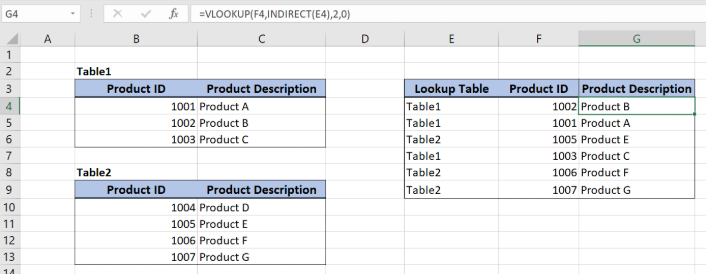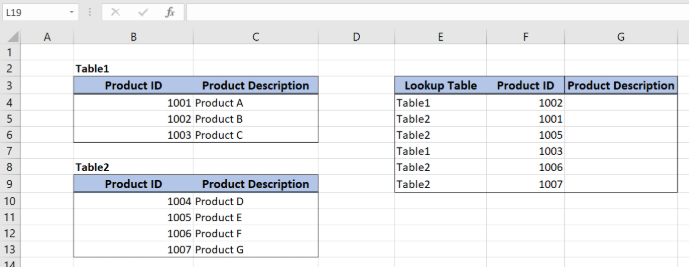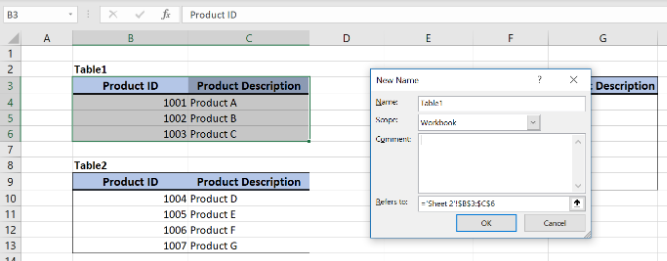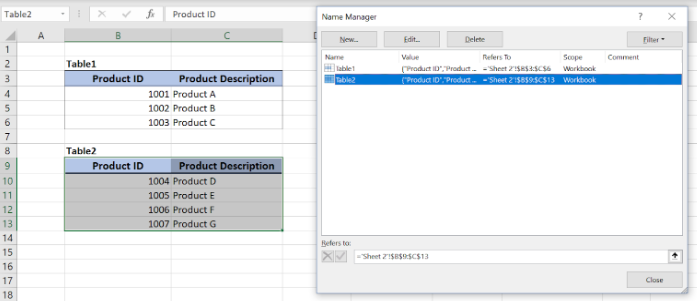Get instant live expert help with Excel or Google Sheets“My Excelchat expert helped me in less than 20 minutes, saving me what would have been 5 hours of work!”

#### Post your problem and you’ll get Expert help in seconds.

Your message must be at least 40 characters
Our professional Expert are available now. Your privacy is guaranteed.

# Dynamic Lookup Table with INDIRECT

While using the VLOOKUP function in Excel, sometimes we need to lookup multiple tables. In order to create the dynamic VLOOKUP formula, we will nest the INDIRECT function inside of a VLOOKUP function to dynamically reference multiple tables within the lookup formula. In this tutorial, we will see how to create a dynamic lookup table with INDIRECT function.Figure 1. Final result

## Syntax of the INDIRECT and VLOOKUP formula

The generic formula for creating a dynamic lookup table:

=VLOOKUP(lookup_value, INDIRECT(text), 2, 0)

The components of the formula are:

• INDIRECT by this Excel function we can reference the specific sheet and the cell range where we want to summarize the data
• VLOOKUP – `=VLOOKUP(lookup_value, table_array, col_index_num, range_lookup)`

The parameters of the VLOOKUP function are:

• lookup_value – a value that we want to find in the VLOOKUP table
• table_array – a range in which we want to lookup
• col_index_num – a column number from which we would like to pull a value
• range_lookup – default value 0. This means that we want to find an exact match for a lookup value.
• Named range – a range of values which we want to sum

## Setting up Our DataFigure 2. Data structure for a dynamic VLOOKUP function

In the ranges B3:C6 and B9:C13, we have two tables with similar structures, but different data values. The tables consist of “Product ID” (column B) and “Product Description” (column C). In the column G, we want to get Product description from the two VLOOKUP tables, based on “Lookup table” and “Product ID” cells values (columns E and F).

To be able to reference dynamically the table, we will first need to create named ranges for both VLOOKUP tables.

## Create Named Ranges

Follow these steps to create named ranges:

• Select the table for which we want to create a named range (B3:C6)
• In Formula tab click on the Name Manager
• In popup screen select New
• A new window will appear and we will have to define “Name”, “Scope” and “Refers to”Figure 3. How to create named range in Name Manager

• Name of the range will be “Table1”, Scope will be the Workbook, while Refers to will be Table1 (B3:C6)
• Just click OK and we created a named range “Table1”
• In order to create the same named range for the other table, just repeat steps above, but first select the range B9:C13.Figure 4. Named ranges for Product tables

• As a result, in Figure 3, we created two named ranges referencing to Product data

## Application of the modified VLOOKUP formula with the INDIRECT function

Our goal is to get Product description in the column G, based on Product ID from the column F. Using the INDIRECT function, we will decide which table to use in VLOOKUP (column E).

The formula looks like:

`=VLOOKUP(F4, INDIRECT(E4), 2, 0)`

In our example, the lookup_value is the cell F4. The parameter table_array. The parameter table_array is the result of INDIRECT(E4) function. Col_index_numhas value 2, as we want to pull value from the second column of the range. Finally, range_lookup has value 0, because we want to find an exact match of “Lookup column” values.

To apply the VLOOKUP with dynamic tables, we need to follow these steps:

• Define named ranges for the tables
• Select cell G4 and click on it
• Insert the formula: `=VLOOKUP(F4, INDIRECT(E4), 2, 0)`
• Press enterFigure 5. Dynamic Lookup table with INDIRECT function

The INDIRECT(E4) function returns “Table1”, which will be table_array parameter in the VLOOKUP function. This means that we want to find Product B (F4) in Table1 (B3:C6). The value of the Product description for 1002 in Table1 is Product B, which is the result of the function in the cell G4.

Most of the time, the problem we will need to solve will be more complex than a simple application of a formula or function. If you want to save hours of research and frustration, try our live Excelchat service! Our Excel Experts are available 24/7 to answer any Excel question you may have. We guarantee a connection within 30 seconds and a customized solution within 20 minutes.

### Did this post not answer your question? Get a solution from connecting with the expert.Another blog reader asked this question today on Excelchat:
Solution examplesI have a pivot table in which per order (on the rows) stands how much products they ordered per size (on the columns). I want to determine which combinations of quantities of sizes people order. And I want to count these combinations.
Solved by E. W. in 60 minsI have 500 numbers in column A with aproximatly 15digits. In column B I have 5 numbers with 6 digits Can a find a formula wich can transfer all numbers from A column wich start with some 6 digitsa from B column
Solved by V. L. in 32 minsI have a sheet with 3 columns. First column is a code for records in column B (has 563 records). What I need is a formula to show me if what is in column C (has 4400 records) is in column B and if it is to take the code for that record. Example: Column A(code corresponding to column B): 12, 14, 15, 19 Column B(names): asd, adf, ade, aqw Column C(names): akd, adf, ade, anb, wgs I need something that would say, if record in column C (for example adf) is matching record in column B (adf) return the code from column A (the code corresponding to that record in column B),if not return blank.
Solved by C. J. in 8 minsIf the data in A matches the data in C, then I need the data in E to be placed in B
Solved by X. W. in 40 minsI have a workbook with 2 sheets. First sheet has column A which is a device name, then columns B - infinity that have numeric "tags" for that device. Each device can have one or 20 "tags", tags are all numerical. Worksheet 2 has column A which is numeric value and B which is a human readable value of what the tag is. Question is, how do I replace all the tag numbers in worksheet 1 with the actual human readable values is sheet 2?
Solved by T. H. in 16 mins## Subscribe to Excelchat.coAnother blog reader asked this question today on Excelchat: